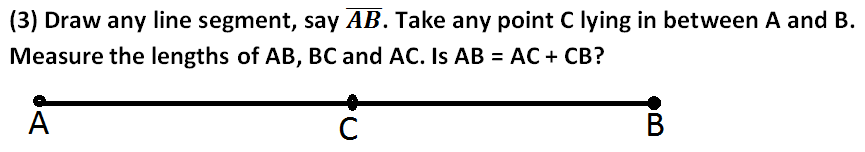# NCERT Class 6 Mathematics Fifth Chapter Understanding Elementary Shapes Exercise 5.1 Solutions

## EXERCISE 5.1

(1) What is the disadvantage in comparing line segments by mere observation?

(2) Why is it better to use a divider than a ruler, while measuring the length of a line segment?(4) If A,B,C are three points on a line such that AB = 5 cm, BC = 3 cm and AC = 8 cm, which one of them lies between the other two?Ans: Point B lies between the A and C.

In case you are missed :- NCERT Solution for Decimals Exercise 8.6(6) If B is the mid point of AC and C is the mid point of BD, where A,B,C,D lie on a straight line, say why AB = CD?Ans: AB = BC and BC = CD; therefore AB = CD

(7) Draw five triangles and measure their sides. Check in each case, if the sum of the lengths of any two sides is always less than the third side.

In case you are missed :- Next Chapter Exercise Solution

Updated: April 14, 2023 — 1:56 pm